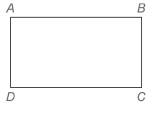Chapter 4.2, Problem 36EElementary Geometry For College St...

7th Edition
Alexander + 2 others
ISBN: 9781337614085

Solutions

Chapter
SectionElementary Geometry For College St...

7th Edition
Alexander + 2 others
ISBN: 9781337614085
Textbook Problem

Are ▱   A B C D and ▱   E F G H congruent if:a) A B ¯ ≅ E F ¯ and B C ¯ ≅ F G ¯ ? b) A B ¯ ≅ E F ¯ , B C ¯ ≅ F G ¯ , and ∠ A ≅ ∠ E ?To determine

a)

Whether ABCD and EFGH congruent when AB¯EF¯ and BC¯FG¯

Explanation

Given,

ABCD and EFGH

AB¯EF¯

BC¯FG¯

Draw the diagonal AC for the ABCD and EG for the EFGH

To determine

b)

Whether ABCD and EFGH congruent when AB¯EF¯, BC¯FG¯ and AE.

Still sussing out bartleby?

Check out a sample textbook solution.

See a sample solution

The Solution to Your Study Problems

Bartleby provides explanations to thousands of textbook problems written by our experts, many with advanced degrees!

Get Started

Evaluate each expression: (8+6)4+8

Elementary Technical Mathematics

Evaluate the indefinite integral. sinxsin(cosx)dx

Single Variable Calculus: Early Transcendentals

True or False: The function graphed at the right is one-to-one.

Study Guide for Stewart's Single Variable Calculus: Early Transcendentals, 8th